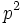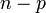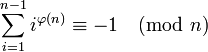Giuga number

Definition

A composite numberis termed a Giuga number if and only if it satisfies the following equivalent conditions:

1. For every prime numberdividing, we have thatdivides, or equivalently, thatdivides.
2. We have the following congruence:wheredenotes the Euler totient function of.

Note that all prime numbers satisfy the stated condition but we deliberately exclude them by imposing the restriction of being composite.